The solutions of Data Interpretation are explained here. To get the Questions, click CAT Data Interpretation questions.

Ultimate Guide to Kickstart your CAT Exam Preparation

#### Solutions for Data Interpretation

1) Option (a)

Solution:

Option (b) is a violation of condition 1.

Option (c) is a violation of condition 3.

Option (d) is a violation of condition 6.

The correct answer is option a.

2) Ans. (b)

Solution: Since he did not paint any happy pink => he won’t be painting any room blue ( condition 3) and he also won’t paint any room violet latte (condition 5) => he won’t paint any room jumping yellow (condition 4). So, he can’t paint 4 types of colours out of 7 => he can paint 3 different types of colours. The correct answer is b.

3) Ans. (d)

Solution: if Mr. X paints a violet latte => he must paint happy pink that too 2 rooms (condition 5 and 6).

If Mr. X paints happy pink => he will paint 2 rooms with it (condition 6) and he can paint 1 violet latte.

If Mr. X paints a room blue => he cannot paint jumping yellow (condition 2), he painted happy pink that too two of them (condition 3 and 6).

He also could have painted a room hazy grey, terry cherry or violet latte as these two have got no constraints on them.

So, option (d) can also be true.

Hence none of these is the answer.

4) Ans. (c)

Solution: From the solution of the 2ns question, he should paint happy pink if he has to paint the maximum colours possible => he cannot paint teal ( condition 1). the correct answer is c.

5) Ans. (b)

Solution: He can paint hazy grey and then he will paint the minimum number of colours 1 as there are no constraints on him for painting hazy grey.

If he paints happy pink, he cannot paint teal and he can paint any one of jumping yellow or blue (conditions 1 and 2). =>maximum number of colours that he can paint = 5. The correct answer is (b).

6) Option (a)

Solution: Go from the answer options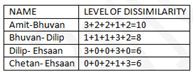7) Based on the table below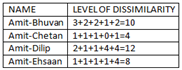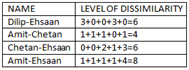9) The person whose second preference is Rose is Bhuvan and the one whose fourth preference is orchid is Dilip.

Their level of dissimilarity is 8 (from table 1)

10) From table two, the answer is 8

11) Option(c)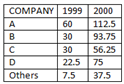Growth in sales of B = 63.75

Growth in sales of A= 52.5

Growth in sales of C= 26.25

Growth in sales of B= 30

So option (c).

12) It will be equal to that of the whole market = 10%

13) The ratio will not change because if the total sales are doubled, the sales of D will also double for both years.

Hence the ratio will be 75/22.5=10/3

14) Bros = 30% of A

A= 30% of detergent market

Bros= 30% of (30% of market)=9% of market.

15) To determine profit percentage, both sales and expenditure are required. As expenditure data is not available, the profit percentage cannot be determined

16) Cost of C = Sale/(100+profit percentage) x 100 = 150×15%/1.25 = 18 crore

C’s cost in 2000= 20

C’s sale in 2000= 75

Profit percentage= 55/20 x 100= 275

Solutions questions 17-19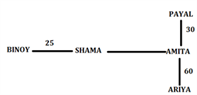17) To the left of Amita is Binoy and Shama, northeast of both is Payal.

18) B-S(25) S-AM(40) AM-ARIYA(60) ARIYA-P(90) TOTAL=215

19) Northeast of Shama- Payal South of Payal- Amita and Ariya

Solutions questions 20-22

This should be solved either by assumption using answer options or by forming equations. Since none of these is also involved, its better to go by using equations

Let y1, y2 … be the indefinite years. Let t be the year blue was born, j green and m- yellow.

Let y be the current year y – 8 – m = ½ ( y2- j) = 1+y3 – ty3 – m = 5( y+2-t)— (1)

y+10 –t= 2( y1-j) y1 –m= 9(y1 –t)————(2)

t+1-m = 3+ y4 – ty4 –j = 3(y5 – 6 – m )y5 –j = ½ (y6 – t) y6 – m =10 + y7 – my7 – j = 1/3 (y8 – t) y8 – m = 3(j-m)

t= y-3j = y-8m = y-15

Therefore, green= 8 years, blue= 3 years & yellow = 15 years

Solutions questions 23-27

Let the marks scored by Charles, David, Hanish, Kedar, Mahoud, Ninja and Raul be represented by C, D, H, K, M, N and R respectively.

From (a), all scored distinct marks:

From (b), C > K > D

From (c), H > M, N > R

From (d), Kedar scored the fourth highest marks.

From (e), M > C

From (c), R is not the least marks scored.

∴ D is the least.

From (b), (c) and (e), H > M > C > K > D

As K is the fourth-highest score and D the lowest score and as N > R, N and R must be the fifth and sixth highest scores respectively.

H > M > C > K > N > R > D

23) Mahoud scored the second-highest marks.

So, Option (b)

24) Raul scored the second-lowest marks.

So, Option (b)

25) Only Hanish and Mahoud scored more marks than Charles.

So, Option (d)

26)
Only Raul scored in between the scores of Ninja and David.

So, Option (c)

27) Only Option (d) is true.

So, Option (d)

28) Total ragi surplus between 98-98 to 00-01 = 6+9+8+12 = 35 lakh tones

Total ragi produced between 97-98 and 00-01 = 132 + 138 +140 + 148 = 558 lakh tones

Percentage of production exported = 35/558 x 100= 6.27%

29) Cumulative production of ragi= 678 lakh tones

Cumulative consumption of rice = 647 lakh tones

Cumulative production exceeds cumulative consumption by 4.7%

30)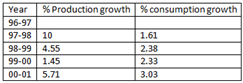From the table, we can see that not in all years in the YOY% growth of production greater that YOY% growth in consumption.

Hence, the statement I is not true Overall production has grown faster than consumption. Hence, statement II is correct

In 99-00, surplus ragi was less than that in 98-99. hence statement III is also not true. Answer= d

31) Production increased from 140 lakh tones to 148 lakh tones, increase 8/140 = 5.71%

32) The year 97-98 alone witnessed a 2 digit rate of growth in production

Solutions questions 33-37

There are 2 cases

(1) D, E, H, B in team X and K, G, F in team Y (total = 40)

So the only possible team Y is K, G, F, L, M, N and I (total=115)

(2) D, E, F in team X and K, G, H, B in team Y (total = 55)

So the possible additions to Y are

(a) L, M and N (total = 53)

(b) L, M and I (total = 60)

(c) I, M and N (total = 55)

(d) I, L and N (total = 57)

33) (d).

34) (b).

35) (a).

36) (c).

37) (d).

38) Option B has no industrialist.

39) (FHAD) (HIBC) (FIAD) (AFDI) (ABFI) (ACHI) (CDHI) (BCGI) (CEDH) – 9 panels

40) I appear on 7 panels.

41) A and B are neither economists nor industrialists. Hence there has to be an economist and an industrialist from RGP if

F is selected➜ I can be selected

if G is selected ➜

I can be selected hence option d Solutions questions 42-46 Tabulate the total number of points obtained by each person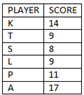From the data in the table, it can be clearly inferred that Anand wins the tournament. Also, the semi-finalists and the finalist can be deduced as K, P and A.

Also, one definite player of the semis is P, and it can also be said that P loses in the semis. Therefore the finals will be played between K and A.

We can directly mark the answers to questions 42-d and 45)-d

If A won the tournament, then he will have a score of 14-3=11 before the finals. Notice that K has only one loss and that loss is the loss in the finals, which implies that K has won all the tournaments before.

Before the finals, A’s score will stand at 14, P’s score at 11 ( he gains one point after losing the semis) and K’s score will stand at 13, who will be the top scorer and will directly go to the finals.

From this, we can clearly say that A will play the semis against P.

A’s score then becomes 11+3=14 and P’s score 10+1=11

With this we can answer questions 43)- d and 44)-c also, 46) option (c)

Only statement 3 is true as K has only one loss, which occurs in the finals

Solutions for questions 47-51

Since there are 5 staff members, if one gets the correct order in which 4 players won the tournament, then definitely the order for all 5 players will be correct. Since it is given that all 5 staff got diff values, it must be 5,3,2,1,0

Assume that Staff A got all of them correct then B, C, D, and E got 1,3,0,2 players in the correct order.

Similarly, checking for other staff members, we arrive at two possibilities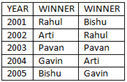47) Option (a).

48) Option (b).

49) In either arrangement, D did not get any of the players in the correct order.

50) Cannot be determined.

51) Since “2” of the winners being in the right order is the middle amount from (5,3,2,1,0) the amount won = (2+1)thousand = 3000 rupees( Even if you are not solving the entire caselet, you must solve this question as in any case the middle value will be 3000).

Solutions questions 52-56:

Calculate the total score by each student and the total score obtained by each student WRT each university as follows (including +5 for those who have done a national level project)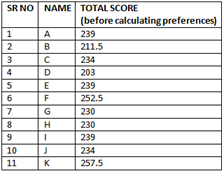Now calculate the score based on preference for each university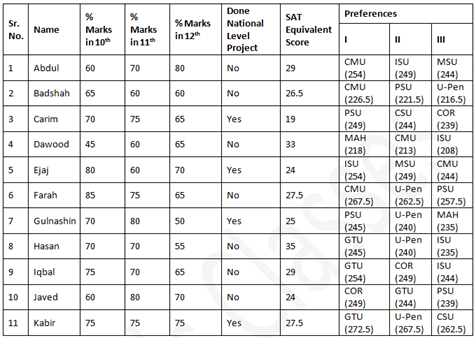The final table can be deduced as follows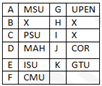Solutions for questions 59 to 63:

From (a), (b) and (c),

as A lives in Delhi, he does not work in S

Also, he does not work in Q and T

From (v), B works in ‘I’ ∴ A works in R

From (d) and (e), none of D, B and E lives in Chennai.

∴ C lines in Chennai.

From (c) and (d), as B works in ‘I’, he does not live in Bangalore.

D lives in Bangalore and works in S.

E works in Q and B lives in Mumbai. The distribution table is as follows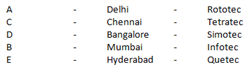59) B lives in Mumbai. (A)

60) D works in Simotec. (C)

61) The person who works in Tetratec lives in Chennai. (B)

62) The person who works in Infotec lives in Mumbai. (C)

63) The person who lives in Hyderabad works in Quetec. (A)

Solutions questions 64-68:

Based on the information, we can start from the bottom-most row of the table and fill it upwards as shown(The loser is highlighted)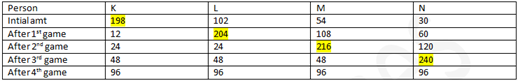So answers are (c), (b),(b) (d) and (d)

69) Two batches have to be formed from students J, K, L, M, N, O, P and Q. If K and L are in one batch, then Q must be in that batch, as K and Q are together.

J cannot be in that batch as it is given that J and K cannot go together.

K, L, Q form a team of 3 members, then the fourth member may be M or N or O or P.

As M and O do not go together, either M alone or O alone should go with K, L, Q.

So the other batch may be either “J, N, O, P” or “J, M, N, P”. Option (C)

70) It is given that A. if Football is selected, then Hockey cannot be selected. B. if Simon selects Tennis, then Jose does not select Football.

Hence if Jose selects Football, Simon cannot select Tennis. C. if Bharath selects Baseball, then Simon cannot select Tennis.

Hence if Simon selects Tennis, Bharath cannot select Baseball.

D. if Jose selects Hockey, then Simon selects Cricket and Football.

Hence if Simon does not select Cricket or does not select Football, then Jose cannot select Hockey.

Simon does not select Tennis, as Bharath selects Baseball. Further, if Football is selected, Hockey cannot be selected.

Therefore, the sports that Simon cannot select now are Hockey and Tennis. Option (A)

Stay tuned with BYJU’S to get the latest updates on CAT Exam. Visit BYJU’S to know the CAT Syllabus, CAT Exam Pattern, etc.